Fundamentals of Financial Management, Concise Edition (MindTap Course List)
9th Edition
ISBN: 9781305635937
Author: Eugene F. Brigham, Joel F. Houston
Publisher: Cengage Learning# The concept of capital budgeting and whether there is any similarity between a firm’s capital budgeting decisions or individual investment decisions. Capital Budgeting: It refers to the long-term investment decisions that have been taken by the top management of a company and that are irreversible in nature. These decisions require an investment of a large amount of cash of the company.

QuestionChapter 11, Problem 24IC

a.

Summary Introduction

## To explain: The concept of capital budgeting and whether there is any similarity between a firm’s capital budgeting decisions or individual investment decisions.Capital Budgeting:It refers to the long-term investment decisions that have been taken by the top management of a company and that are irreversible in nature. These decisions require an investment of a large amount of cash of the company.

a.

Expert Solution

• Capital budgeting can be referred to the long-term investment decisions that have been taken by the top management of a company and that are irreversible in nature. These decisions require an investment of a large amount of cash of the company.
• Capital budgeting is also an investment decision like any individual investment decision but the only difference is that it includes a greater amount of investment in comparison to individual’s investment.

### Explanation of Solution

• Capital budgeting is the long-term investment decisions which includes a great amount of investment and that are irreversible in nature, unlike an individual’s investment decision.
• Capital budgeting and individual’s investment are similar in all the aspects but they differ in the amount to be invested or the size of the project or investment.
Conclusion

Thus, capital budgeting is also an investment decision like individual’s investment decision but greater in size than that.

b.

Summary Introduction

### To explain: The difference between mutually exclusive and independent projects and between normal and nonnormal cash flow projects.

b.

Expert Solution

• The difference between the mutually exclusive projects and independent projects is that in case of the mutually exclusive project the acceptance or rejection of a project affects the decision regarding acceptance or rejection of the other project which is not there in case of independent projects.
• The difference between the normal and nonnormal cash flow projects is that in case of normal cash flows there are only one or two cash outflows at the beginning of the project and after that only cash inflows would be there which is not there in case of nonnormal cash flow project.

### Explanation of Solution

• Mutually exclusive projects are those in which acceptance or rejection of one project will automatically lead to a decision regarding another project while in case of independent project acceptance or rejection of one project will not lead to a decision regarding another project.
• In case of mutually exclusive projects, only one project can be selected while in case of independent projects more than one project can be selected.
• Normal cash flows projects are those in which there are one or two cash outflows at the beginning of the project and after that only cash inflows arrive while in cash of nonnormal cash flow projects cash outflow can occur in the middle of the life of the project.
• Normal cash flow projects include the series of cash inflows while in case of nonnormal cash flow project there is no such series.
Conclusion

Thus, there is a huge difference between mutually exclusive and independent project and between normal and nonnormal cash flow projects.

c.

1.

Summary Introduction

c.

1.

Expert Solution

### Explanation of Solution

NPV refers to the method under capital budgeting which includes the calculation of the net present value of the project in which company is investing. The calculation is done by calculating the difference between the value of cash inflow and value of cash outflow after taking into consideration the discounted rate.

Project L

Given,

Cost of the project is $100 thousand dollars. Cash inflow at the end of the 1st year is$10 thousand dollar.

Cash inflow at the end of the 2nd year is $60 thousand dollar. Cash inflow at the end of the 3rd year is$80 thousand dollar.

Life of the project is 3 years.

WACC of the project is 10%.

Calculation of NPV for project L on the spreadsheet is,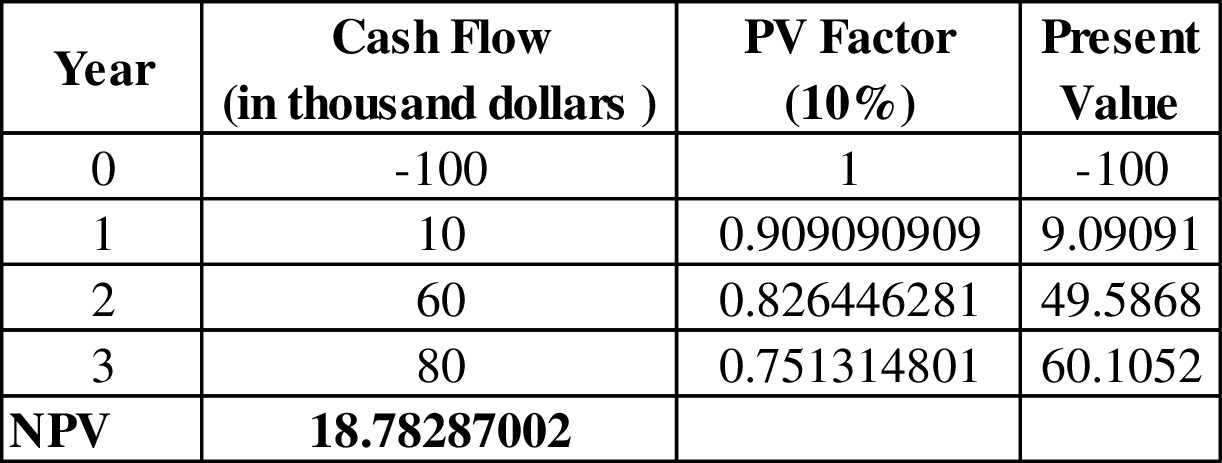Table (1)

The NPV of project L is $18.78 thousand dollars. Project S Given, Cost of the project is$100 thousand dollars.

Cash inflow at the end of the 1st year is $70 thousand dollar. Cash inflow at the end of the 2nd year is$50 thousand dollar.

Cash inflow at the end of the 3rd year is $20 thousand dollar. Life of the project is 3 years. WACC of the project is 10%. Calculation of NPV for project S on the spreadsheet is,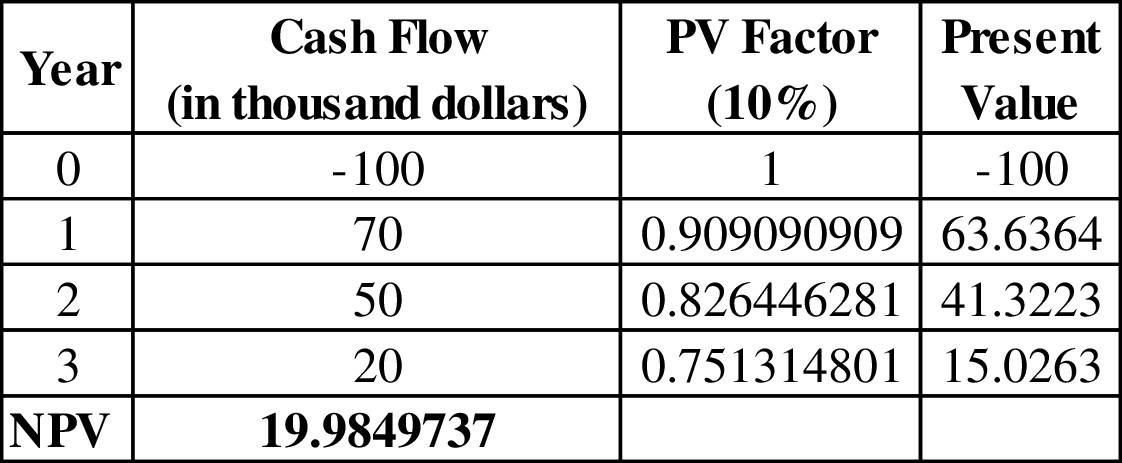Table (2) The NPV of project S is$19.98 thousand dollars.

• NPV can be defined as the method for decision making related to capital budgeting investment decisions. It is a method that provides the profit to be generated by the project after considering the time value of money.
• The rule under NPV method for decision making is higher the NPV, the higher would be the profit from the project would be.
• In NPV method a project is accepted when NPV is greater than 0 and it is rejected when it is less than 0 and at 0 NPV the project may or may not be selected.
Conclusion

Thus, NPV of project L is $18.78 thousand dollars and NPV of project S is$19.98 thousand dollars.

2.

Summary Introduction

### To explain: The rationale behind NPV method and whether project L or S should be selected if they independent and when they are mutually exclusive.

2.

Expert Solution

The rationale behind NPV is that the 0 NPV of a project implies that the project is generating exact amount that the company is needed to pay for the project as debt and NPV greater than 0 shows that the company is generating profit after paying for its debt of the project while NPV less than 0 shows that project would not be able to generate the amount even to pay for its debt.

If the projects are independent then the company should accept both the projects as both are having NPV greater than 0.

If projects are mutually exclusive than the company should accept project S over project L as project S is having higher NPV than project L.

### Explanation of Solution

• Since in case of independent projects, multiple projects can be selected at a time, and both projects are having positive NPV, both projects can be accepted as they are independent.
• Since in case of the mutually exclusive project only one project can be selected and projects S has higher NPV than project L, project S is better than project L and it should be accepted over project L.
Conclusion

Thus, if the projects are independent than a project with highest positive NPVcan be accepted and if the projects are mutually exclusive than project S should be accepted over project L.

3.

Summary Introduction

### To explain: Whether the NPV changes with the change in WACC.

3.

Expert Solution

Yes, the NPV changes with the change in WACC.

### Explanation of Solution

• The NPV is totally dependent on the value of the WACC as NPV considers the discounted cash flows instead of normal cash flows.
• The NPV of a project and WACC has a direct relationship with each other which means an increase in WACC would increase the value of NPV of the project and vice versa.
Conclusion

Thus, the NPV changes with the change in WACC.

d.

1.

Summary Introduction

d.

1.

Expert Solution

### Explanation of Solution

IRR refers to the rate of return that is computed by the company to make a decision of selection of a project for investment. This rate provides the basis for selection of projects with a lower cost of capital and rejection of project with a higher cost of capital.

Project L

Given,

Cost of the project is $100 thousand dollars. Cash inflow at the end of the 1st year is$10 thousand dollar.

Cash inflow at the end of the 2nd year is $60 thousand dollar. Cash inflow at the end of the 3rd year is$80 thousand dollar.

Life of the project is 3 years.

WACC of the project is 10%.

Calculation of IRR for project L on the spreadsheet is: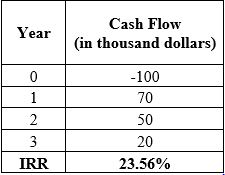Table (3)

The IRR of project L is 18.13%.

Project S

Given,

Cost of the project is $100 thousand dollars. Cash inflow at the end of the 1st year is$70 thousand dollar.

Cash inflow at the end of the 2nd year is $50 thousand dollar. Cash inflow at the end of the 3rd year is$20 thousand dollar.

Life of the project is 3 years.

WACC of the project is 10%.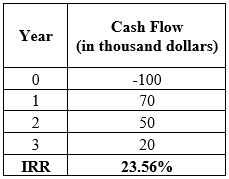Table (4)

The IRR of project S is 23.56%.

• IRR can be defined as the rate of return that is to be achieved from the project by the end of the life of the project.
• It is that rate of return at which NPV stands 0 which means this is the minimum rate of return that a company should get from a project after accepting it.
Conclusion

Thus, IRR of project L is 18.13% and IRR of project S is 23.56%.

2.

Summary Introduction

### To explain: The relationship of IRR on a project to the YTM on a bond.

2.

Expert Solution

The relationship of IRR on a project is same as the relationship of YTM that is yield to maturity on a bond as both provides the minimum rate of return that the investment should provide after accepting the project or bond.

### Explanation of Solution

• YTM is the minimum rate of return that a bond should provide after investing in it below which the investment would incur loss instead of profit as in the case of IRR at which NPV stands 0.
• IRR is the expected rate of return on a project while the YTM is promised a rate of return on the bond.
Conclusion

Thus, the relationship of IRR on a project is same as the relationship of YTM that is yield to maturity on a bond.

3.

Summary Introduction

### To explain: The logic behind the IRR method and whether the project L or S should be selected when they are an independent project and when they are mutually exclusive projects.

3.

Expert Solution

The logic to calculate IRR is to know the project’s rate of return and to know whether the project is generating economic profit or an economic loss.

If the projects are independent then both projects can be accepted as both provide IRR greater than the cost of capital.

If the projects are mutually exclusive then project S should be accepted over project L as the IRR of project S is higher than project L.

### Explanation of Solution

• Since in case of independent projects, multiple projects can be selected at a time, and both projects are having IRR greater than the cost of capital both projects should be accepted.
• Since in case of the mutually exclusive project, only one project can be selected and projects have higher IRR than project L, project S is better than project L and it should be accepted over project L.
Conclusion

Thus, both projects must be selected when they are independent and project S should be accepted over project L when they are mutually exclusive projects.

4.

Summary Introduction

### To explain: Whether the project IRR change with the change in WACC.

4.

Expert Solution

No, the project’s IRR does not get changed with the change in WACC.

### Explanation of Solution

• The IRR of a project is independent of WACC as it does not consider the cash flows after discounting them, so WACC has no impact on IRR.
• The IRR of a project remains same for a project at the different level of WACC.
Conclusion

Thus, IRR does not change with the change in WACC.

e.

1.

Summary Introduction

e.

1.

Expert Solution

### Explanation of Solution

The NPV profile of project L and project S is: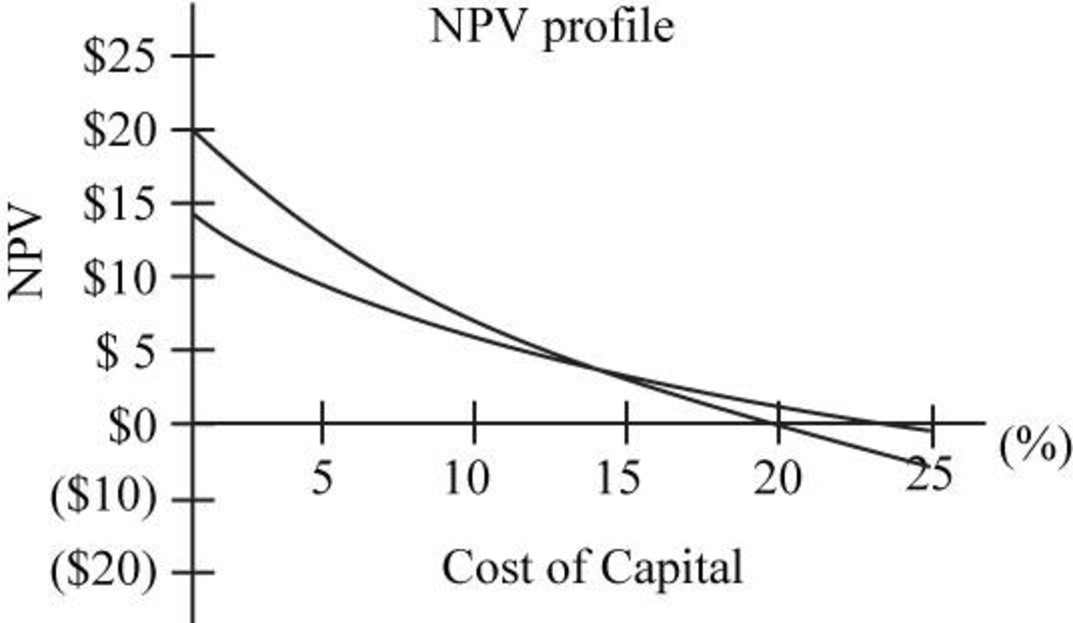Fig.1

In the above figure, NP profile of project L is given by the blue line and of project S is given by black line.

• In the above figure, it can be seen that NPV of project L is decreasing much faster than project S with the increase in WACC.
• From the above figure, it can be seen that the NPV of both the project stands equal to approximately 9% WACC, so the crossover rate is 9%.
Conclusion

Thus, the crossover rate of the two projects is 9%.

2.

Summary Introduction

### To explain: Whether to accept project A or project B on the basis of NPV profile when they are independent or when they are mutually exclusive.

2.

Expert Solution

• When projects are independent then both projects can be selected when WACC is less than 18% and only project S should be accepted when it is between 18 to 24%.
• When the projects are mutually exclusive then project L should be chosen over project S when WACC is less than crossover rate and when it is greater than crossover rate project S should be chosen over project L.

### Explanation of Solution

• From the above figure it can be seen that NPV of project L is positive when the WACC is less than 18% and NPV of project S is positive when WACC is less than 24%, so when the projects are independent both projects can be accepted when WACC is less than 18% and only project S should be accepted when it is between 18 to 24%
• From the above figure it can be seen that the NPV of project L is higher when the WACC is less than the crossover rate and after that NPV of project S is higher, so, when the projects are mutually exclusive the project L should be accepted when WACC is less than the crossover rate and project S should be accepted when WACC is between crossover rate and 24%
Conclusion

Thus, on the basis of NPV profile, the selection of projects totally depends upon the rate of WACC whether they are independent or mutually exclusive.

f.

1.

Summary Introduction

### To explain: The underlying cause of ranking conflicts between IRR and NPV.

f.

1.

Expert Solution

The major cause of ranking conflict between IRR and NPV is that they have different assumptions for reinvestment rate of cash flows. There are other few reasons too which are:

• Difference between the size of the projects, which have a direct effect on NPV of the project but might not have any effect on IRR.
• The difference in the timing of the projects which is considered in NPV but not in IRR.

### Explanation of Solution

• Since NPV assumes the reinvestment rate as the cost of capital of the project while IRR considers the reinvestment at IRR; it is the major cause of conflict between the ranking between the NPV and IRR.
• Since NPV is affected by the size of the project, the greater the size of the project the greater would be the NPV which is not there in case of IRR, this also creates the conflict between NPV and IRR.
• Since NPV considers the time value of money, so it gets affected with the increase in time of the project which is not there in case of IRR, so this also creates the conflict between IRR and NPV.
Conclusion

Thus, in case of mutually exclusive projects, there can be a conflict between the rankings of IRR and NPV.

2.

Summary Introduction

### To explain: the reinvestment rate assumption and the way it affects the NPV versus IRR conflicts.

2.

Expert Solution

Reinvestment rate assumption is referred to the assumption related to the discounting or compounding the cash flows generated through a project.

It increases the conflict between the NPV and IRR due to the difference in the assumption regarding reinvestment rate.

### Explanation of Solution

• In case of NPV, the discounting of the cash flows is done on the cost of capital, so it considers the reinvestment of cash flows at the cost of capital.
• In case of IRR, the compounding of the cash flows is done on the IRR, so it assumes the reinvestment of cash flows at the IRR.
• This difference in assumption increases the conflict between IRR and NPV.
Conclusion

Thus, the assumption regarding reinvestment rate increases the conflict between IRR and NPV.

3.

Summary Introduction

### To explain: Whether the NPV or IRR is a better method.

3.

Expert Solution

NPV is a better method than IRR.

### Explanation of Solution

• Since NPV assumes the reinvestment at cost of capital which is more logical and appropriate than IRR; it is a better method than IRR for an investment decision.
• Since NPV also considers the shareholders’ wealth which is not there in case of IRR, so, NPV is better than IRR.
• There is a uniquesolution in case of NPV for each project at different WACC while IRR can have multiple solutions.
Conclusion

Thus, NPV is a better method than IRR.

g.

1.

Summary Introduction

g.

1.

Expert Solution

### Explanation of Solution

MIRR refers to the rate of return that is computed by the company to make a decision of selection and ranking of a project for investment. This is a modified version of IRR with reinvestment of cash flows at the cost of capital.

Project L

Given,

Cost of the project is $100 thousand dollars. Cash inflow at the end of the 1st year is$10 thousand dollar.

Cash inflow at the end of the 2nd year is $60 thousand dollar. Cash inflow at the end of the 3rd year is$80 thousand dollar.

Life of the project is 3 years.

WACC of the project is 10%.

Calculation of IRR for project L on the spreadsheet is: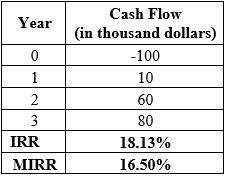Table (5)

The MIRR of project L is 16.50%.

Project S

Given,

Cost of the project is $100 thousand dollars. Cash inflow at the end of the 1st year is$70 thousand dollar.

Cash inflow at the end of the 2nd year is $50 thousand dollar. Cash inflow at the end of the 3rd year is$20 thousand dollar.

Life of the project is 3 years.

WACC of the project is 10%.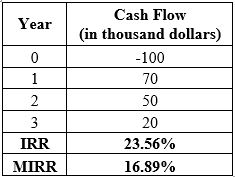Table (6)

The MIRR of project S is 16.89%.

• The MIRR can be defined as the rate of return which is expected by the company to be earned at the end of the life of the project.
• It is a modified version of IRR which assumes the reinvestment at cost of capital in spite of IRR.
Conclusion

Thus, the MIRR of project L is 16.50% and of project, S is 16.89%.

2.

Summary Introduction

### To explain: The advantages and disadvantages of MIRR as compared to NPV.

2.

Expert Solution

The advantages of MIRR as compared to NPV are:

• It can provide a higher value for a project which has low NPV with the same cost of capital.
• It includes the future value of cash flow that is to be expected in spite of the present value of future cash flow as in case of NPV.

The disadvantages of MIRR as compared to NPV are:

• It does not consider the shareholders’ wealth as in the case of NPV.
• It can provide multiple solutions for one project.

### Explanation of Solution

• MIRR is the modified rate of return which includes the calculation of the future values of the given cash flow which is not there in case of NPV.
• MIRR can provide a greater ranking to the project which has such low NPV.
• MIRR does not consider the shareholders’ wealth as in case of NPV which is a major disadvantage of MIRR.
• Unlike NPV, MIRR can have multiple solutions of a project which does not provide accurate decision making.
Conclusion

Thus, in spite of advantages, as compared to NPV, MIRR is still not that good as NPV.

h.

1.

Summary Introduction

h.

1.

Expert Solution

### Explanation of Solution

Payback period refers to the time period that is required to get an amount invested in a project with some return on it. In other words, it is the time that a project takes to repay the amount invested with some return attached to it.

Project L

Given,

Cost of the project is $100 thousand dollars. Cash inflow at the end of the 1st year is$10 thousand dollar.

Cash inflow at the end of the 2nd year is $60 thousand dollar. Cash inflow at the end of the 3rd year is$80 thousand dollar.

Life of the project is 3 years.

WACC of the project is 10%.

The formula to calculate payback period is,

PaybackPeriod=(YearofLastNegativeCumulativeCashFlow)+|LastNegativeCumulativeCashflow|(PositiveCashFlowintheNextYear)

Substitute 2 for the year of last negative cumulative cash flow, $30 for last negative cumulative cash flow and$80 for positive cash flow in the next year.

PaybackPeriod=(2)+|$30|($80)=2+0.375=2.375

The payback period of the project L is 2.375 years.

Working note:

Calculation of cumulative cash flow,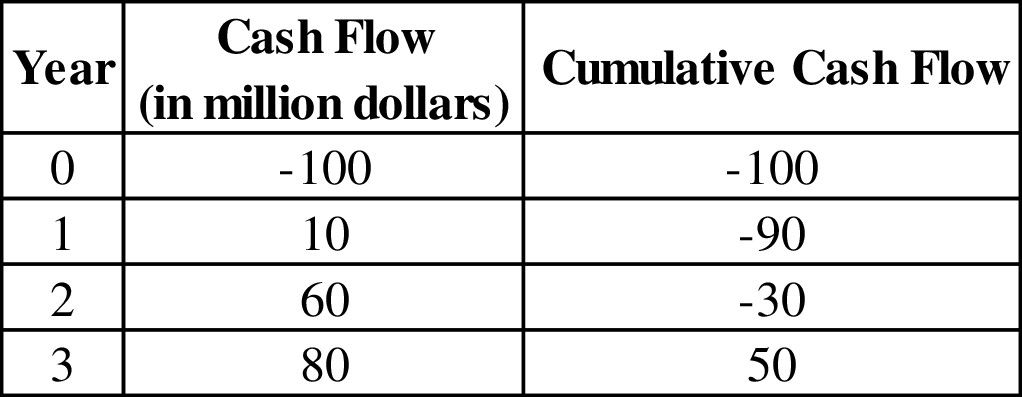Table (7)

Project S

Given,

Cost of the project is $100 thousand dollars. Cash inflow at the end of the 1st year is$70 thousand dollar.

Cash inflow at the end of the 2nd year is $50 thousand dollar. Cash inflow at the end of the 3rd year is$20 thousand dollar.

Life of the project is 3 years.

WACC of the project is 10%.

Substitute 1 for the year of last negative cumulative cash flow, $30 for last negative cumulative cash flow and$50 for positive cash flow in the next year.

PaybackPeriod=(1)+|$30|($50)=1+0.6=1.6

The payback period for the project S is 1.6 years.

Working note:

Calculation of cumulative cash flow,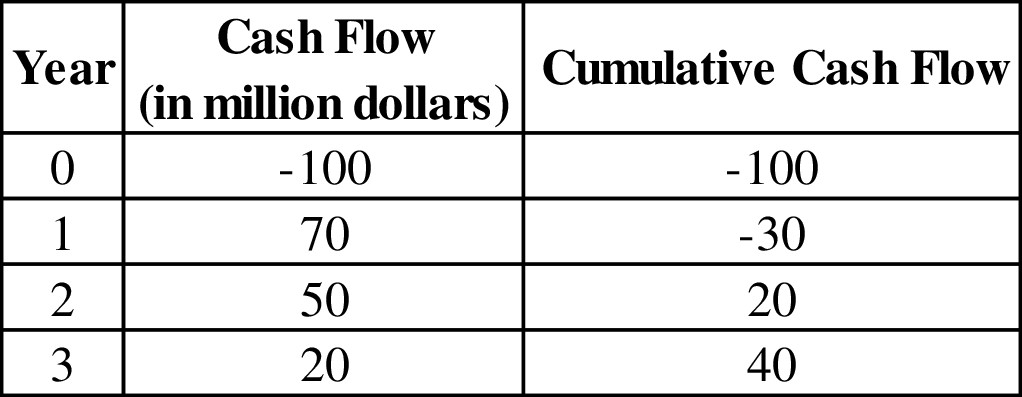Table (8)

• The payback period can be defined as the period after which the return can be expected.
• It does not consider the time value of money, so normal cash flows are considered in the calculation in spite of discounted cash flows.
Conclusion

Thus, the payback period of project L is 2.375 years and project S is 1.6 years.

2.

Summary Introduction

2.

Expert Solution

### Explanation of Solution

The rationale behind the payback period is that when the payback period of the project is lesser than the company decided payback period, it is accepted and when it is greater than the company decided payback period, it is rejected.

When the payback period is 2 years and projects are independent, then project S should be chosen as the payback period of project S is lesser than 2 years.

When the payback period is 2 years and projects are mutually exclusive, then project S should be chosen as the payback period of project S is lesser than 2 years.

• In case of independent projects multiple projects can be chosen at a time but as only project, S has lesser time period than 2 years, so only project S would be selected.
• In case of mutually exclusive projects one project can be selected at a time and since project S has lesser time period than 2 years, so only project S would be selected.
Conclusion

Thus, project S should be chosen over project L on the basis of payback period whether the projects are independent or mutually exclusive.

3.

Summary Introduction

### To explain: The difference between regular payback period and discounted payback period.

3.

Expert Solution

The only difference between the payback period and the discounted payback period is that the payback period does not consider the time value of money while the discounted payback period assumes the time value of money.

### Explanation of Solution

• The payback period is the period after which the return on investment is expected by the company on the normal cash flows while the discounted payback period is the period after which the return on investment is expected on discounted cash flows.
• Payback period does not consider the time value of money while discounted payback period considers the time value of money.
Conclusion

Thus, the difference between the payback period and the discounted payback period is the treatment of time value of money.

4.

Summary Introduction

### To explain: The two disadvantages of discounted payback period and whether payback period is useful in capital budgeting.

4.

Expert Solution

The two disadvantages of discounted payback period are:

• It does not consider the cash flow after the payback period.
• It does not take into consideration the shareholders’ wealth.

Payback period is not much use for the company for capital budgeting decision.

### Explanation of Solution

• Discounted payback period does contemplate the time value of money but it does consider the amount of cash flow generated after the payback period which is its major disadvantage.
• Discounted payback period does not consider the shareholders’ wealth which is there isa case of NPV. So, the discounted payback period is not much use for a company to make capital budgeting decisions but it is still calculated to have estimated time period for return.
Conclusion

Thus, the payback period is not a much useful method for capital budgeting decisions.

i.

1.

Summary Introduction

i.

1.

Expert Solution

### Explanation of Solution

Given information:

Cost of the project is $0.8 million dollars. Cash inflow at the end of the 1st year is$5.0 million dollars.

Cash outflow at the end of the 2nd year is \$5.0 million dollars.

Life of the project is 2 years.

Cost of capital is 10%.

Calculation of NPV, IRR,and MIRR of project P on the spreadsheet is,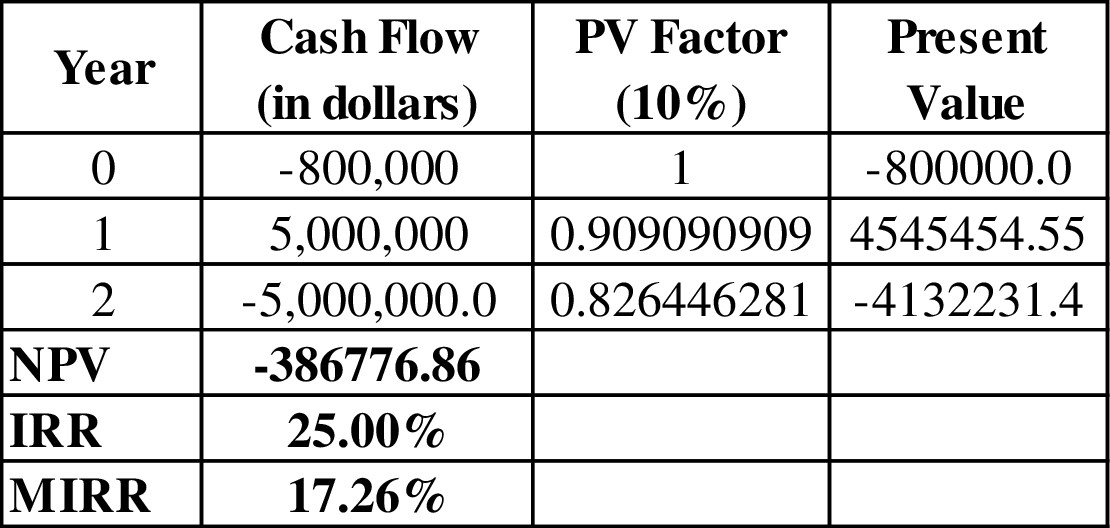Table (9)

The NPV, IRR, and MIRR of project P are-386,776.86 dollars, 25%, 17.26% respectively.

Conclusion

Thus, the NPV, IRR, and MIRR of project P are-386,776.86 dollars, 25%, and 17.26% respectively.

2.

Summary Introduction

### To explain: Whether the project p has normal or nonnormal cash flows and whether the project P should be accepted or not and construct NPV profile for project P.

2.

Expert Solution

The NPV profile of project P is: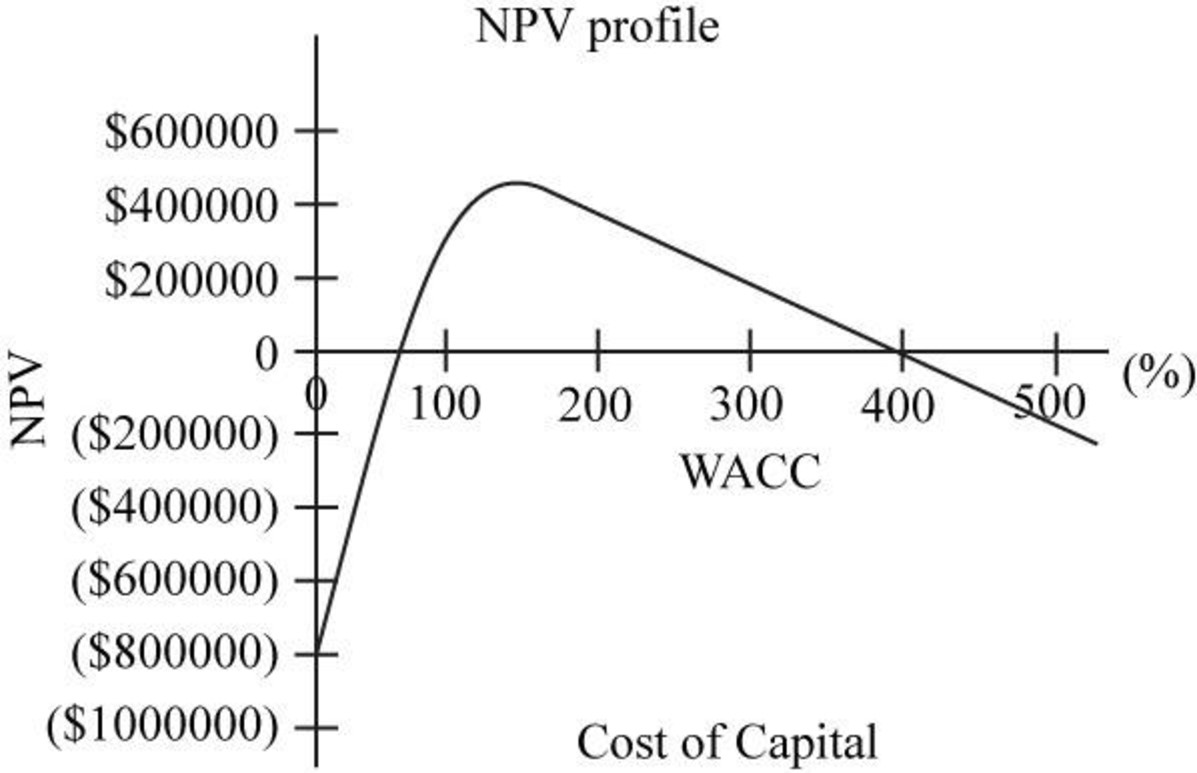Fig.2

The project P has nonnormal cash flows.

The project P should not be accepted.

### Explanation of Solution

From the above figure it can be seen that project with WACC less than 50% provides negative NPV and after 50% of WACC, it provides positive NPV.

Since the project P does not have the series of cash inflow; it provides non-normal cash flows in which there is no series of positive cash inflow.

Since the NPV of project P at 10% WACC is negative, so it should not be accepted by the company.

Conclusion

Thus, the project P has non-normal cash flow and it should not be accepted.

### Want to see more full solutions like this?

Subscribe now to access step-by-step solutions to millions of textbook problems written by subject matter experts!Fundamentals of Financial Management, Concise Edition (MindTap Course List)
9th Edition
ISBN: 9781305635937
Author: Eugene F. Brigham, Joel F. Houston
Publisher: Cengage Learning